# Samacheer Kalvi Books: Tamilnadu State Board Text Books Solutions

## Samacheer Kalvi 11th Computer Science Chapter 10 Flow of Control Notes PDF Download: Tamil Nadu STD 11th Computer Science Chapter 10 Flow of Control NotesSamacheer Kalvi 11th Computer Science Chapter 10 Flow of Control Notes PDF Download: Tamil Nadu STD 11th Computer Science Chapter 10 Flow of Control Notes

## Samacheer Kalvi 11th Computer Science Chapter 10 Flow of Control Notes PDF Download

We bring to you specially curated Samacheer Kalvi 11th Computer Science Chapter 10 Flow of Control Notes PDF which have been prepared by our subject experts after carefully following the trend of the exam in the last few years. The notes will not only serve for revision purposes, but also will have several cuts and easy methods to go about a difficult problem.

 Board Tamilnadu Board Study Material Notes Class Samacheer Kalvi 11th Computer Science Subject 11th Computer Science Chapter Chapter 10 Flow of Control Format PDF Provider Samacheer Kalvi Books

## How to Download Samacheer Kalvi 11th Computer Science Chapter 10 Flow of Control Notes PDFs?

2. Click on the Samacheer Kalvi 11th Computer Science Notes PDF.
3. Look for your preferred subject.
4. Now download the Samacheer Kalvi 11th Computer Science Chapter 10 Flow of Control notes PDF.

## Download Samacheer Kalvi 11th Computer Science Chapter 10 Flow of Control Chapterwise Notes PDF

PART – 1

Question 1.
What is the alternate name of null statement?
(a) No statement
(b) Empty statement
(c) Void statement
(d) Zero statement
(b) Empty statement

Question 2.
In C++, the group of statements should enclosed within:
(a) { }
(b) []
(c) ()
(d) <>
(a) { }

Question 3.
The set of statements that are executed again and again in iteration is called as:
(a) condition
(b) loop
(c) statement
(d) body of loop
(d) body of loop

Question 4.
The multi way branching statement:
(a) if
(b) if … else
(c) switch
(d) for
(c) switch

Question 5.
How many types of iteration statements exist?
(a) 2
(b) 3
(c) 4
(d) 5
(b) 3

Question 6.
How many times the following loop will execute? for (int i = 0; i < 10; i++)
(a) 0
(b) 10
(c) 9
(d) 11
(b) 10

Question 7.
Which of the following is the exit control loop?
(a) for
(b) while
(c) do … while
(d) if … else
(c) do … while

Question 8.
Identify the odd one from the keywords of jump statements:
(a) break
(b) switch
(c) goto
(d) continue
(a) break

Question 9.
A loop that contains another loop inside its body:
(a) Nested loop
(b) Inner loop
(c) Inline loop
(d) Nesting of loop
(a) Nested loop

PART – 2
II. Answers to all the questions

Question 1.
What is a null statement and compound statement?
Null statement:
The “null or empty statement” is a statement containing only a semicolon. It takes the following form:
;// it is a null statement.
Null statement is commonly used as place holders in iteration statements or as statements on which to place labels at the end of compound statements or functions.

Compound statement:
C++ allows a group of statements enclosed by pair of braces { }. This group of statements is called as a compound statement or a block.
The general format of compound statement is: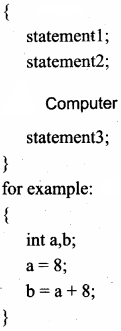Question 2.
What is a selection statement? Write its types.
The selection statement means the statements are executed depends upon a condition. If a condition is true, a true block is executed otherwise a false block is executed. This statement is also called a decision statement or selection statement.
Types:

1. if… else statement
2. switch statement

Question 3.
Correct the following code segment: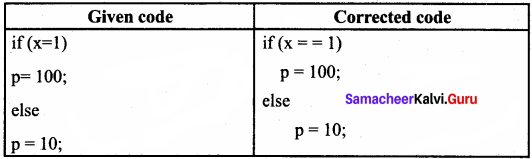Question 4.
What will be the output of the following code: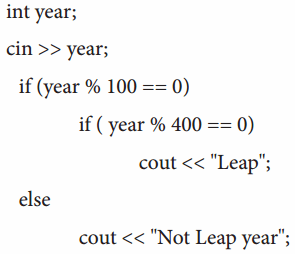If the input is given is

1. 2000
2. 2003
3. 2010

1. Output
(Input = 2000)
Leap

2. Output
(Input = 2003)
Not leap Year

3. Output (Input = 2010)
Not leap Year

Question 5.
What is the output of the following code?
for (int i = 2; i < = 10 ; i + = 2)
cout<<i;
Output:
2 4 6 8 10

Question 6.
Write a for loop that displays the number from 21 to 30.
For loop to display numbers from 21 to 30:
for(i = 21; i<=30;i++)
cout<< i ;

Question 7.
Write a while loop that displays numbers 2,4, 6, 8 ……………… 20.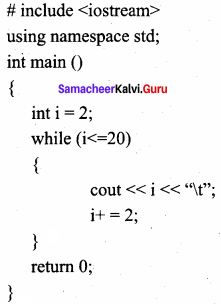Output:
2 4 6 8 10 12 13 14 16 18 20

Question 8.
Compare an if and a? : operator.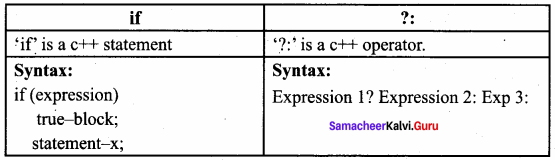PART – 3 III.
III. Answers to all the questions Question

Question 1.
Convert the following if – else to a single conditional statement: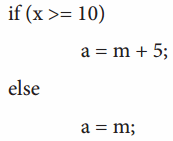a = (x> =10)? m + 5:m;

Question 2.
Rewrite the following code so that it is functional: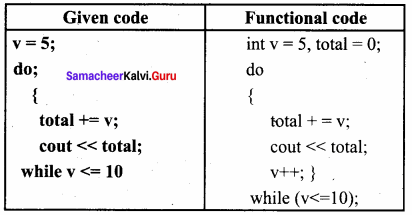Question 3.
Write a C++ program to print the multiplication table of a given number.
PROGRAM
using namespace std;
#include
#include
int main()
{
int n;
cout<<“\nEnter Table Number”; cin>>n;
for(int i=l;i<=20;i++)
cout<<setw(2)<<i<<“X”<<n<<” = “<<setw(3)<<i*n<<endi;
return 0;
}

Question 4.
Write the syntax and purpose of the switch statement.
The switch statement is a multi-way branch statement. It provides an easy way to dispatch execution to different parts of code based on the value of the expression. The switch statement replaces multiple if-else sequences.
The syntax of the switch statement is;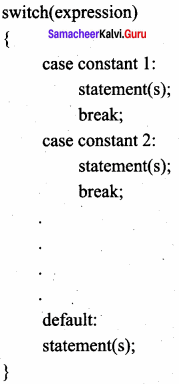Question 5.
Write a short program to print the following series:
(a) 1 4 7 10 …… 40
# include
using namespace std;
int main ()
{

cout << “\n PRINT SERIES” ;
for (int i = 1; i< 40; i = i + 3)
cout << i << “1+”;
cin.get();
return ();
}

PART – 4
IV. Answers to all the questions

Question 1.
Explain the control statement with a suitable example.
Control statements are statements that alter the sequence of flow of instructions.
Types of control statements:
1. Selection statement:
The selection statement means the statement (s) executed, depends upon a condition. If a condition is true, a true block (a set of statements) is executed otherwise a false block is executed. This statement is also called a decision statement or selection statement because it helps in making a decision about which set of statements are to be executed.
Example:

1. If
2. Switch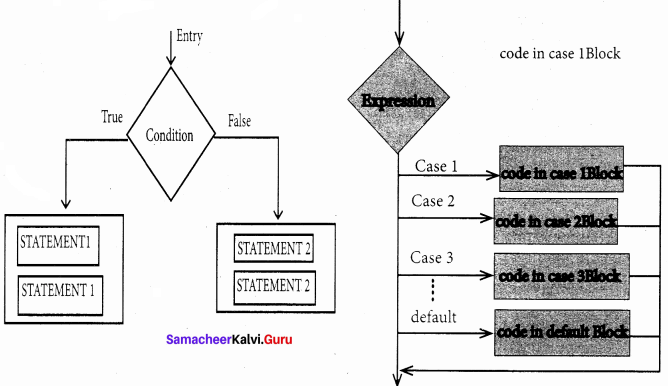2. Iteration (or looping) statement:
An iteration (or loop) is a sequence of one or more statements that are repeatedly executed until a condition is satisfied. These statements are also called control flow statements. It is used to reduce the length of code to reduce the time to execute the program and takes less memory space.

Example:

1. While
2. do ….. while
3. for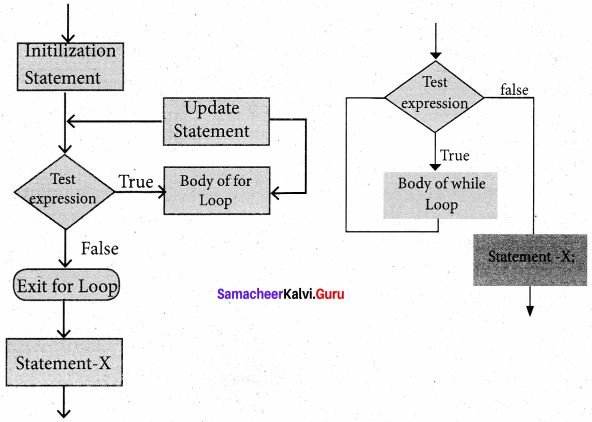Question 2.
What is the entry control loop? Explain any one of the entry control loops with a suitable example.
An entry control loop checks the condition at the time of entry and if condition or expression becomes true then control transfers into the body of the loop, for loop and while loop is examples of Entry Controlled Loop. A while loop is a control flow statement that allows the loop statements to be executed as long as the condition is true.
Type: Entry control loop
Syntax: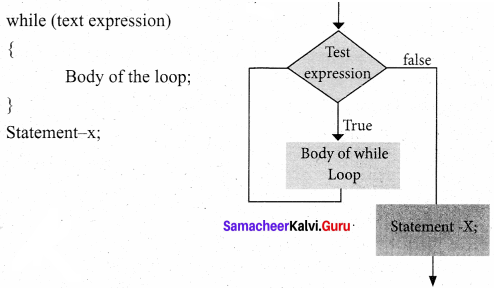Control flow:
Step 1: Test – expression is evaluated to either True or False;
Step 2: If test – expression is true;
(a) The body of the loop is executed.
(b) Control is transferred to step 1.
Step 3: If the test – expression is false, the control exits the while loop.
Example:
int a = 1;
while (a<=10)
{

cout << a << ‘\t+’;
a+=2;

}

Output:
1 3 5 7 9

Question 3.
Write a program to find the LCM and GCD of two numbers.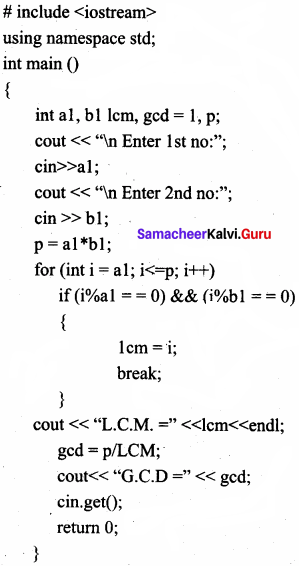Question 4.
Write programs to find the sum of the following series:
(a) x – x22! + x33! + x44! + x55!x66!
(b) x + x22 + x33 + ……… + xnn
(a)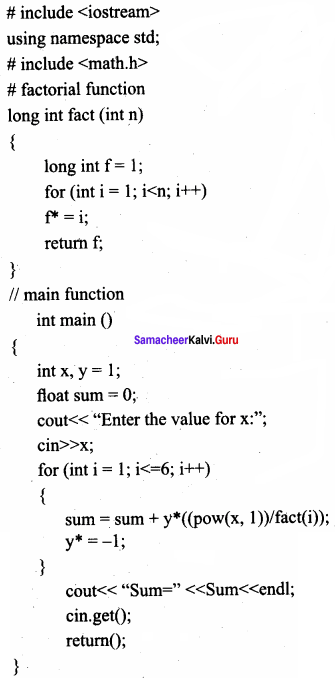(b) # include
using namespace std;
# include int main()
{

int M, L;
float sum = 0;
cout << “Enter the value for M:”; cin >> M;
cout << “Enter the number of terms”; cin >> L;
for (int i = 1; i<=m; i++)
sum = Sum + Pow(m,i)/i;
cout << “Sum=” << Sum << endl;
cin.get();
return 0;

}

Question 5.
Write a program to find sum of the series
S = 1 + x + x2 + ………….. + xn
# include
using namespace std;
# include int main()
{

int x, n;
float sum = 0;
cout << “Enter the value for x:”; cin >> x;
cout << “Enter the number of terms”; cin >> n;
for (int i = 0; i<=n; i++)
sum = Sum + Pow(x,i);
cout << “Sum=” << Sum << endl;
cin.get( );
return 0;

}

### Samacheer Kalvi 11th Computer Science Flow of Control Additional Questions and Answers

PART – 1

Question 1.
Program statements that cause jumps are called as ‘ ……………….
a) Control flow
b) Null statement
c) Compound statement
d) None of these
a) Control flow

Question 2.
Selection statement is also called as ………………..
(a) Decision statement
(b) Sequence statement
(c) Null statement
(d) Compound statement
(a) Decision statement

Question 3.
A (n) ……………. is a set of statements or instructions to perform a specific task.
a) Program
b) Language
c) Algorithm
d) Pseudocode
a) Program

Question 4.
In C++ any non – zero is iterated as true ……………….. and zero is treated as false.
(a) positive numbers
(b) negative numbers
(c) prime numbers
(d) none of these
(b) negative numbers

Question 5.
In a program, the action may be ………………..
a) Decision Making
b) Looping
c) Both A and B
d) None of these
c) Both A and B

Question 6.
Syntax of the conditional operator is ………………..
(a) expression 1? expression 2: expression 3
(b) expression 1: expression 2
(c) expression 1! expression 2: expression 3
(d) expression 1: expression 2: expression 3
(a) expression 1? expression 2: expression 3

Question 7.
…………….. statements are commonly used as placeholders in iteration statements or as statements on which to place labels at the end, of compound statements or functions.
a) Decision making
b) Sequential
c) Loop
d) Null
d) Null

Question 8.
When a switch is a part of the statement sequence of another switch, then it is called as ………………..
(b) Switch statement
(c) Nested switch statement
(d) Empty statement
(c) Nested switch statement

Question 9.
In C++, the group of statements is called a ……………
a) Compound statement
b) Block
c) Null statement
d) Either A or B
d) Either A or B

Question 10.
Every loop has ……………….. elements that are used for different purposes.
(a) 3
(b) 4
(c) 5
(d) 2
(b) 4

Question 11.
In a program, statements may be executed …………..
a) Sequentially
b) Selectively
c) Iteratively
d) Either A or B or C
d) Either A or B or C

PART – 2

Question 1.
Define Control flow.
The flow of control jumps from one part of the code to another segment of the code. Program statements that cause such jumps are called “Control flow”.

Question 2.
Write a program to check whether a person is eligible to vote using if statement.
C++ program to check whether a person is eligible to vote using if statement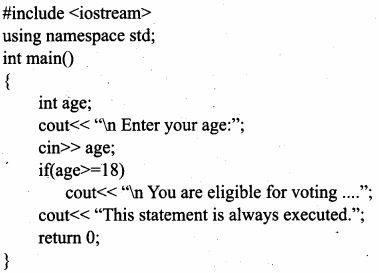Output:
You are eligible for voting…
This statement is always executed.

Question 3.
What are the kinds of statements used in C++?
There are two kinds of statements used in C++.

• Null statement
• Compound statement

Question 4.
Write the syntax for the if-else ladder.
The syntax of the if-else ladder: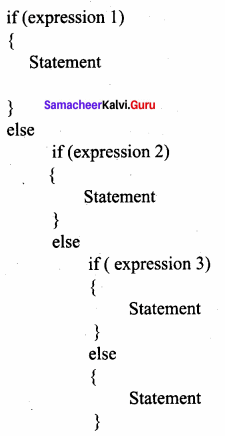Question 5.
What is a nested switch statement?
When a switch is a part of the statement sequence of another switch, then it is called a nested switch statement. The inner switch and the outer switch constant may or may not be the same.

Question 6.
What are the iteration statements in C++?
C++ supports three types of iteration statements. They are:

• for statement
• while statement
• do-while statement

Question 7.
Write a C++ program to sum the numbers from 1 to 10.
C++ program to sum the numbers from 1 to 10 using for loop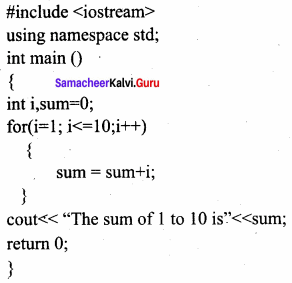Output:
The sum of 1 to 10 is 55

Question 8.
Rewrite the given program.
C++ program to sum the numbers from 1 to 10 using for loop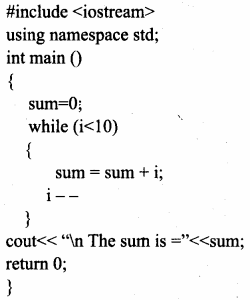C++ program to sum the numbers from 1 to 10 using for loop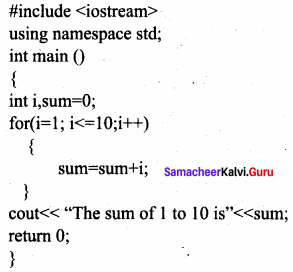Output:
The sum of 1 to 10 is 55

Question 9.
What are jump statements? Give its types.
Jump statements are used to interrupt the normal flow of the program. Types of jump statements are:

1. go to statement
2. break statement
3. continue statement

Question 10.
What will be the output of the following code.
int a, b, largest;
cout << “\n Enter any two numbers:”; cin >> a >> b;
largest = (a > b)? a:b;
cout << “\n Largest number:” << largest;
return 0;
Output:
Enter any two numbers:
5 10
Largest number: 10

PART – 3
III. Explain in Brief

Question 1.
What are sequence statements?
The sequential statement is the statements that are executed one after another only once from top to bottom. These statements do not alter the flow of execution. These statements are called sequential flow statements. They always end with a semicolon (;).

Question 2.
Write the syntax of the nested switch statement.
The syntax of the nested switch statement is; switch (expression)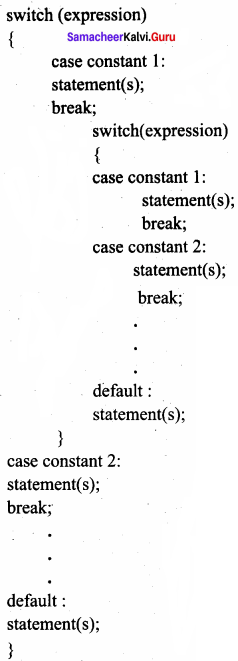Question 3.
Write short notes on Test expression.
The test expression is an expression or condition whose value decides whether the loop – the body will be executed or not. If the expression evaluates to true (i.e., 1), the body of the loop is executed, otherwise, the loop is terminated. In an entry – controlled loop, the test – expression is evaluated before entering into a loop whereas in an exit – controlled loop, the test – expression is evaluated before exiting from the loop.

Question 4.
What is a do-while loop? Write its syntax.
The do-while loop is an exit-controlled loop. In a do-while loop, the condition is evaluated at the bottom of the loop after executing the body of the loop. This means that the body of the loop is executed at least once, even when the condition evaluates false during the first iteration.
The do-while loop syntax is:
do {
Body of the loop;
} while(condition);

Question 5.
What are the differences between break and continue statements?
Break:

• The break is used to terminate the execution of the loop.
• It breaks the iteration.
• When this statement is executed, control will come out from the loop and executes the statement immediately after the loop.
• The break is used with loops as well as switch cases.

Continue:

• Continue is not used to terminate the execution of the loop.
• It skips the iteration.
• When this statement is executed, it will not come out of the loop but moves/jumps to the next iteration of the loop.
• Continue is only used in loops, it is not used in switch cases.

Question 6.
What are the important things to know about the switch statement?
There are some important things to know about the switch statement. They are

1. A switch statement can only work for the quality of comparisons.
2. No two case labels in the same switch can have identical values.
3. If character constants are used in the switch statement, they are automatically converted to their equivalent ASCII codes.
4. The switch statement is a more efficient choice than if in a situation that supports the nature of the switch operation.

PART – 4
IV. Explain in Detail

Question 1.
What are the key differences between if-else and switch statements?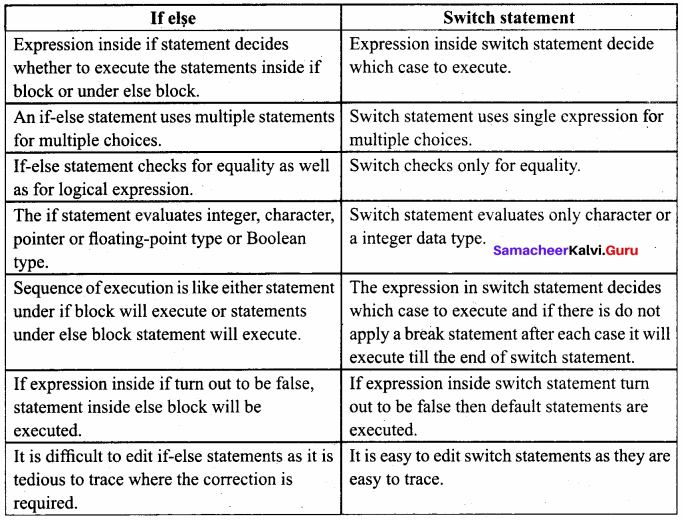Question 2.
Explain about parts of a loop.
Every loop has four elements that are used for different purposes. These elements are:

1. Initialization expression
2. Test expression
3. Update expression
4. The body of the loop

1. Initialization expression(s): The control variable(s) must be initialized before the control enters into a loop. The initialization of the control variable takes place under the initialization expressions. The initialization expression is executed only once at the beginning of the loop.

2. Test Expression: The test expression is an expression or condition whose value decides whether the loop-body will be executed or not. If the expression evaluates to true (i.e., 1), the body of the loop is executed, otherwise, the loop is terminated.

In an entry-controlled loop, the test – expression is evaluated before entering into a loop whereas in an exit-controlled loop, the test – expression is evaluated before exiting from the loop.

3. Update expression: It is used to change the value of the loop variable. This statement is executed at the end of the loop after the body of the loop is executed.

4. The body of the loop: A statement or set of statements forms a body of the loop that is executed repetitively. In an entry-controlled loop, first, the test expression is evaluated and if it is nonzero, the body of the loop is executed otherwise the loop is terminated. In an exit-controlled loop, the body of the loop is executed first then the test-expression is evaluated. If the test – expression is true the body of the loop is repeated otherwise loop is terminated.

Question 3.
Explain jump statements.
Jump statements are used to interrupt the normal flow of the program. Types of Jump Statements are:

1. goto statement
2. break statement
3. continue statement

The goto statement is a control statement which is used to transfer the control from one place to another place without any condition in a program.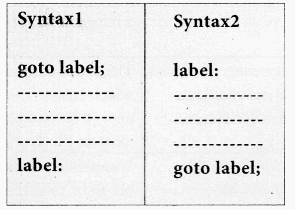A break statement is a jump statement which terminates the execution of the loop and the control is transferred to resume normal execution after the body of the loop.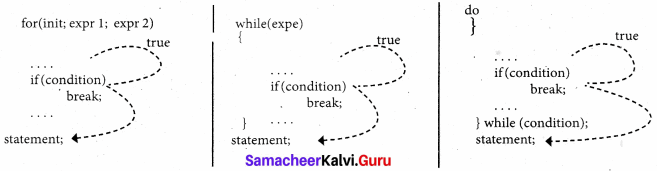The continue statement works quite similar to the break statement. Instead of terminating the loop (break statement), the continue statement forces the loop to continue or execute the next iteration. When the continue statement is executed in the loop, the code inside the loop following the continue statement will be skipped and the next iteration of the loop will begin.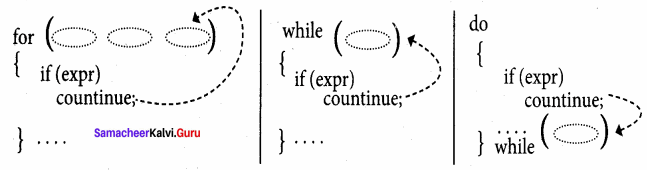Question 4.
Programs to produce the following design using nested loops.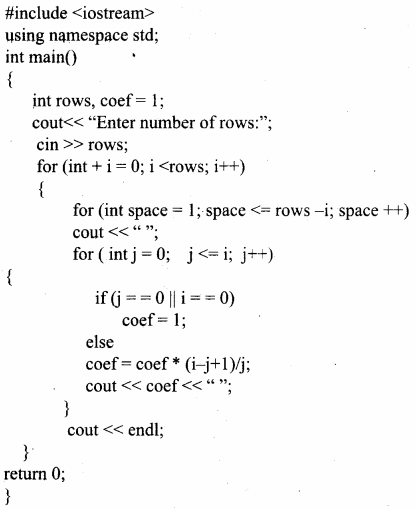Output
Enter number of rows: 5
1
1 1
1 2 1
1 3 3 1
1 4 6 4 1

## How to Prepare using Samacheer Kalvi 11th Computer Science Chapter 10 Flow of Control Notes PDF?

Students must prepare for the upcoming exams from Samacheer Kalvi 11th Computer Science Chapter 10 Flow of Control Notes PDF by following certain essential steps which are provided below.

• Use Samacheer Kalvi 11th Computer Science Chapter 10 Flow of Control notes by paying attention to facts and ideas.
• Pay attention to the important topics
• Refer TN Board books as well as the books recommended.
• Correctly follow the notes to reduce the number of questions being answered in the exam incorrectly
• Highlight and explain the concepts in details.

## Frequently Asked Questions on Samacheer Kalvi 11th Computer Science Chapter 10 Flow of Control Notes

#### How to use Samacheer Kalvi 11th Computer Science Chapter 10 Flow of Control Notes for preparation??

Read TN Board thoroughly, make separate notes for points you forget, formulae, reactions, diagrams. Highlight important points in the book itself and make use of the space provided in the margin to jot down other important points on the same topic from different sources.

#### How to make notes for Samacheer Kalvi 11th Computer Science Chapter 10 Flow of Control exam?

Read from hand-made notes prepared after understanding concepts, refrain from replicating from the textbook. Use highlighters for important points. Revise from these notes regularly and formulate your own tricks, shortcuts and mnemonics, mappings etc.
Share: